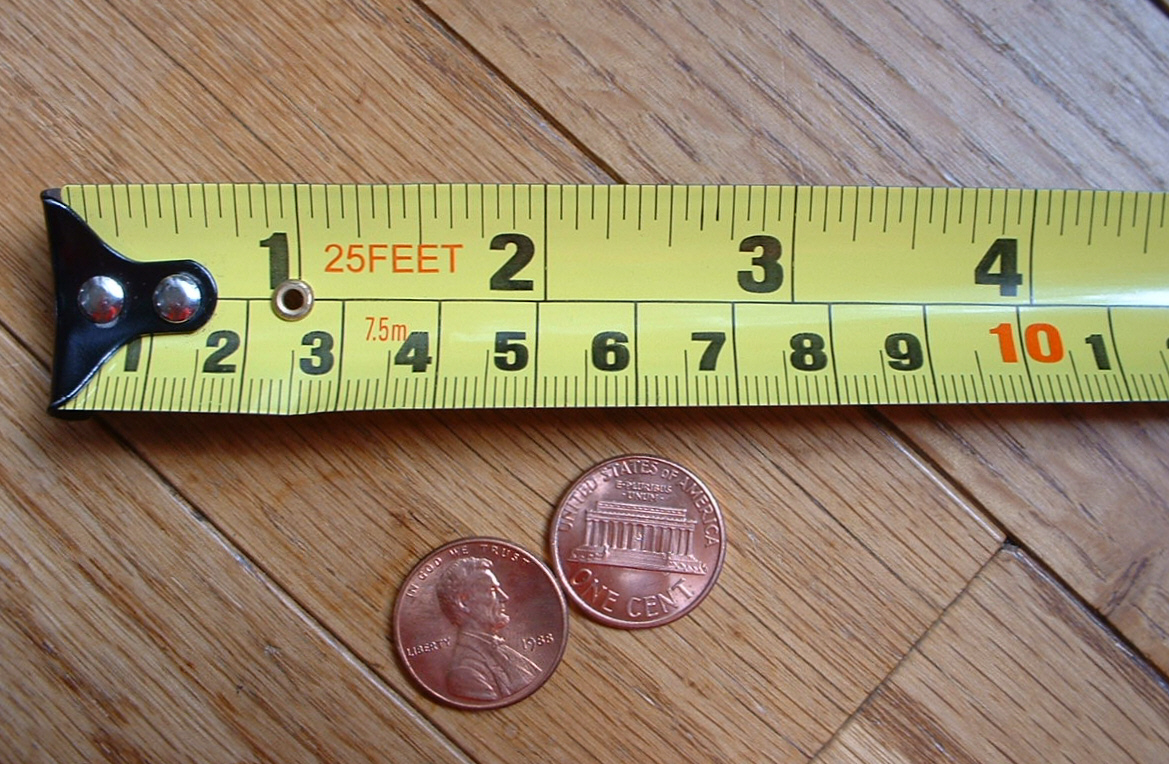# Measurement and Measurement Units in Physics

Measurement is a process of detecting an unknown physical quantity by using standard quantity. For example: Take a book and use ruler (scale) to find its length. Suppose the length was 20 cm. You underwent through a process called Measurement where:

• The unknown physical quantity was the length of book.
• The ruler was the standard quantity.
• 20 was the magnitude.
• cm was the unit of the book-length.## Units of Measurement

Units provide specific meaning to the magnitude of a substance. Units of measurement provide standard to identify measurement of a physical quantify. For example: If you say that, the volume of your notebook is 25, it provides no exact meaning because it could be 25 mm3 or 25 cm3 or 25 dm3 and many more. But if you use units cm3, it provides accurate meaning that the volume of the notebook is 25 cm3.

### Systems of Unit of Measurement

There are different standard and unit of system used in the word. Few common system of measurements are:

CGS unit system

In CGS unit system, length is measured in centimeter, mass is measured in gram and time is measured in second.

Length Mass Time
centimeter gram second

FPS unit system

In FPS system, length is measured in foot, mass is measured in pound and time is measured in second.

Length Mass Time
foot pound second

MKS unit

In MKS system, length is measured in meter, mass is measured in kilogram and time is measured in second.

Length Mass Time
meter kilogram second

### SI unit

Different units are used in different countries for the measurement of physical quantities. In US, pound metrics is used commonly for indicating mass but in India, kilogram is used. To remove this differences, SI (International System of units) system was standardized in 1960. In SI unit,

Name Abbreviation Measure
meter m Length
kilogram kg mass
second s tim
ampere A electric current
Kelvin K thermodynamic temperature
mole mol amount of substance
candela cd luminous intensity

## Divisions of units

### Fundamental Units (Basic Units)

Fundamental units are those units that can express themselves without the assistance of any other units. For example: Kilogram (kg) is a fundamental unit because it is independently expressed and cannot be broken down to multiple units.

### Derived Units

Derived units are those units which cannot be expressed in the absence of fundamental units. For example: Newton (N) is a derived unit because it cannot be expressed in the absence of fundamental unit (meter) and can be broken down to multiple units (Newton equals to kg*m /s2).